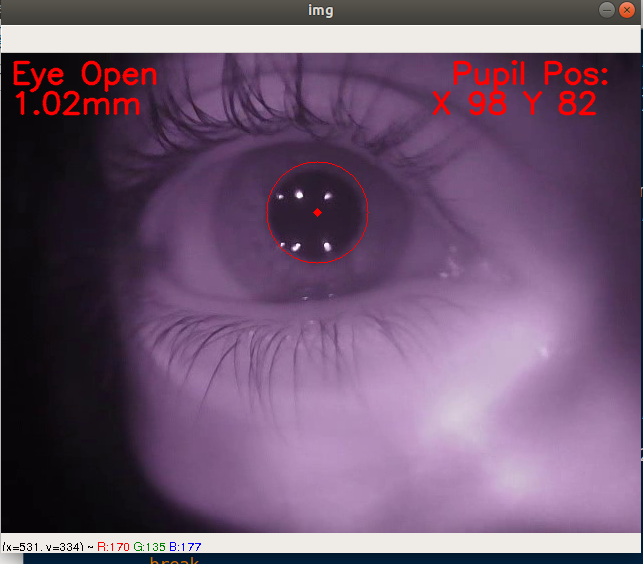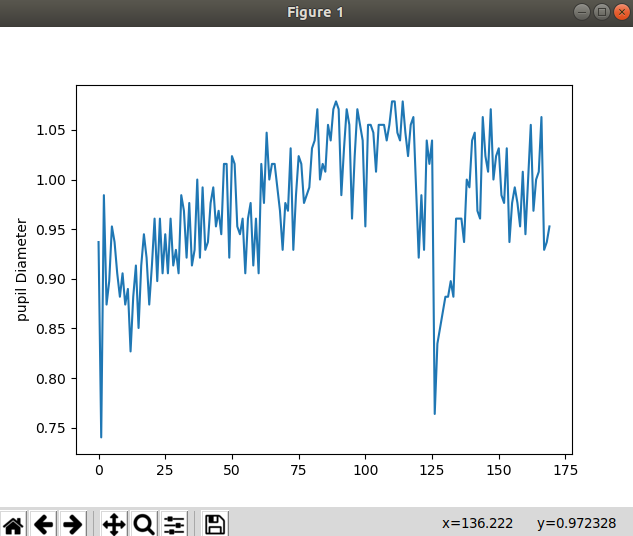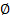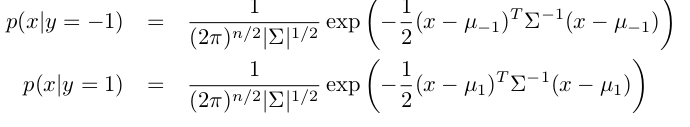## Pupil Detection in Python using OpenCV

##### In this part of Learning Python we Cover Video Processing In Python
Written by |07-Jul-2019 | 0 Comments | 1111 Views

We were doing a project on pupil detection in this lecture we will continue the project where we left off previously in video processing. Now we have to proceed as follows:

` else:`
`            if (blink==False):`
`                blink=True`
`                if blink==True:`
`                     cv2.putText(img,"Blink",(10,90), font, 1,(0,0,255),2,cv2.LINE_AA)`
`            a="Eye Close"`
`            cv2.putText(img,a,(10,30), font, 1,(0,0,255),2,cv2.LINE_AA)`
`            `
`        cv2.imshow('img',img)`
`        k = cv2.waitKey(30) & 0xff`
`        if k == 27:`
`            break`
`    plt.plot(diameter)`
`    plt.ylabel('pupil Diameter')`
`    plt.show()        `
`    cap.release()`
`    cv2.destroyAllWindows()`
`except:`
`    plt.plot(diameter)`
`    plt.ylabel('pupil Diameter')`
`    plt.show()   `

SourceCode Credit: Hassan Rehman, Pupil-Detection, (2018), GitHub repository, https://github.com/HassanRehman11/Pupil-Detection/blob/master/PupilDetection.py

We made some condition for blinking, and in last we will show the image, and when the video is stopped, it will show the diameter plot on Matplotlib.

The final result is as follows:Figure 1

Figure 1 shows that the tracking pupil and showing all the information that we have mentioned. When the video is close it will plot the diameter of the pupil as follows:Figure 2

The full code is as follows:

`import numpy as np`
`import cv2`
`import matplotlib.pyplot as plt`
`import matplotlib.animation as animation`
`eye_cascade = cv2.CascadeClassifier('haarcascae_eye.xml')`
`cap = cv2.VideoCapture('Video.mp4')`

`diameter=[]`
`blink =False`
`bcount = -1`
`kernel = np.ones((5,5),np.uint8)`
`global a`
`font = cv2.FONT_HERSHEY_SIMPLEX`
`try:`
`    while 1:`
`        ret, img = cap.read()`
`        gray = cv2.cvtColor(img, cv2.COLOR_BGR2GRAY)`
`        eyes = eye_cascade.detectMultiScale(gray,1.1,7)`
`        if (len(eyes)>0):`
`            a = "Eye Open"`
`            `
`            if (blink==True):`
`                blink=False`
`               `
`            cv2.putText(img,a,(10,30), font, 1,(0,0,255),2,cv2.LINE_AA)`
`            `
`            for (ex,ey,ew,eh) in eyes:`
`                #cv2.rectangle(img,(ex,ey),(ex+ew,ey+eh),(0,255,0),2)`
`                roi_gray2 = gray[ey:ey+eh, ex:ex+ew]`
`                roi_color2 = img[ey:ey+eh, ex:ex+ew]`
`                blur = cv2.GaussianBlur(roi_gray2,(5,5),10)`
`                erosion = cv2.erode(blur,kernel,iterations = 2)`
`                ret3,th3 = cv2.threshold(erosion,0,255,cv2.THRESH_BINARY+cv2.THRESH_OTSU)`
`                circles = cv2.HoughCircles(erosion,cv2.HOUGH_GRADIENT,4,200,param1=20,param2=150,minRadius=0,maxRadius=0)`
`                try:`
`                    for i in circles[0,:]:`
`                        if(i>0 and i<55):`
`                            cv2.circle(roi_color2,(i,i),i,(0,0,255),1)`
`                            cv2.putText(img,"Pupil Pos:",(450,30), font, 1,(0,0,255),2,cv2.LINE_AA)`
`                            cv2.putText(img,"X "+str(int(i))+" Y "+str(int(i)),(430,60), font, 1,(0,0,255),2,cv2.LINE_AA)`
`                            d = (i/2.0)`
`                            dmm = 1/(25.4/d)`
`                            diameter.append(dmm)`
`                            cv2.putText(img,str('{0:.2f}'.format(dmm))+"mm",(10,60), font, 1,(0,0,255),2,cv2.LINE_AA)`
`                            cv2.circle(roi_color2,(i,i),2,(0,0,255),3)`
`                            #cv2.imshow('erosion',erosion)`
`                except Exception as e:`
`                    pass`
`                `
`        else:`
`            if (blink==False):`
`                blink=True`
`                if blink==True:`
`                     cv2.putText(img,"Blink",(10,90), font, 1,(0,0,255),2,cv2.LINE_AA)`
`            a="Eye Close"`
`            cv2.putText(img,a,(10,30), font, 1,(0,0,255),2,cv2.LINE_AA)`
`            `
`        cv2.imshow('img',img)`
`        k = cv2.waitKey(30) & 0xff`
`        if k == 27:`
`            breakwhich datasets suits the most`
`    plt.plot(diameter)`
`    plt.ylabel('pupil Diameter')`
`    plt.show()        `
`    cap.release()`
`    cv2.destroyAllWindows()`
`except:`
`    plt.plot(diameter)`
`    plt.ylabel('pupil Diameter')`
`    plt.show()   `

SourceCode Credit: Hassan Rehman, Pupil-Detection, (2018), GitHub repository, https://github.com/HassanRehman11/Pupil-Detection/blob/master/PupilDetection.py

Here we are done with OpenCV, and now we will see some machine learning concept. It is straightforward to code the machine learning algorithm but there are lots of machine learning algorithm, and the main task is how different dataset is for the different algorithm.

Learning Algorithms

• Generative Learning Algorithms

• Discriminative Learning Algorithms

Generative Learning Algorithm:

Unlike another learning algorithm like logistic regression where the decision is made based on the boundary line. Suppose we have to identify apple from orange. We will make a model that classifies them and create a boundary between them. The clear difference indicates the difference between apple and orange. Whether in generative learning algorithm the model is trained by first looking on apple how apple looks like and then looking on an orange how it looks like. Finally, to classify the unknown fruit we first look against apple and then orange and then compare the probability of both occurrences.

Suppose the input data is x e {1,2} and for x, y e {0,1} and the value of x and y is {(1,0), (1,0), (2,0), (2,1)}

The empirical measure of p(x|y) will be:

 Y=0 y=1 x=1 1/2 0 x=2 1/4 1/4

And empirical measure for p(y|x)

 Y=0 y=1 x=1 1 0 x=2 1/2 1/2

And it is called probability distribution and is given by

p(y|x) = p(x|y) p(y) / p(x)

by Gaussian discriminant analysis we can elaborate it as if:

p(y) = {if y =1, 1-if y= -1}Another example of the generative learning algorithm is Naive Bayes Algorithm.

Naive Bayes Algorithm:

Naive Bayes algorithm is a probabilistic classifier on which Bayes Theorem is applied with strong independence assumption between the features.

In plain English we can write the formula of Naive Bayes probability as follows:

probability = prior x likelihood / evident

mathematically we represent it as:

p(y|x) = p(x|y) p(y) / p(x) which is joint probability distribution.

Uses:

Naive Bayes algorithm can be used in the following predictions:

• Spam Email Filtration
• Match Winning Predictions
• Gender Predictions

Spam Filtration:

Spam filtering is a broader classification as it involves text classification. As we know that the predictive model can’t recognize the text as a feature vector. For that, we have to first email as spam or not spam as 0 and 1. The words that are spam, we have to make a dictionary assigning them an Id.

Suppose we have a feature vector of length 5000 than the equation of finding the probability can be represent it as:

The feature vector x for a given y will be:

p (x1,……..,x5000 | y)

=  p(x1 | y) p(x2 | y) p(x3 | y) p(x4 | y) ……….p(x5000 | y)

= i=1np(x,y)